# Thermodynamics

## Homework Help & Tutoring

We offer an array of different online Thermodynamics tutors, all of whom are advanced in their fields and highly qualified to instruct you.Send your subject help request Submit your homework problem, or a general tutoring request.
Get quotes from qualified tutors Receive a response from one of our tutors as soon as possible, sometimes within minutes!
Thermodynamics Tutors Available Now
63 tutors available
Thermodynamics Homework Library
130 total solutions
See what our students are saying
Can you help me with my homework in less than 24 hours?
Can you help me with my exam/quiz/test?
How much will it cost?
What kind of payments do you accept?

# Thermodynamics

Students who study thermodynamics often find it to be one of the most challenging subjects, especially at more advanced levels where calculus is an integral part of the topic.  It is, however, an important topic in both Physics and Chemistry and is something that all physical scientists, engineers, and even many biologists study.

Thermodynamics is the study of Heat and Energy.  Many people use the terms "heat," "energy," and "temperature" interchangeably, but heat is actually one type of energy and temperature represents another type of energy.

Energy Transformation

The first law of thermodynamics is that energy cannot be created or destroyed.  While this law is always true, energy can be transformed from one type to another.  Heat can be changed into kinetic (moving) or latent/potential (stored) energy.  Thermodynamics uses P-V-T (pressure, volume, and temperature) diagrams to model these transformations.

Heat Transfer

Heat can also be transferred from one object to another. This transfer can occur in one of three ways: conduction (by touching), convection (through a fluid), or radiation (as E&M Waves).  Thermodynamics studies these transfers on a macroscopic (object to object) scale and a molecular (atom to atom) scale.  Thermodynamics incorporates the mathematics involved in each of these types of heat transfer including Newton's Law of Cooling and the Stefan-Boltzmann and Wien Laws.

Some of the topics you can expect to see in more detail in your Thermodynamics course:

*  The work-energy theorem

*  Conservation of energy

*  Thermal expansion

*  The ideal gas law

*  Entropy, free energy, and enthalpy

*  Open and closed systems

*  Temperature and kinetic theory

*  Equilibrium

*  Different types of thermodynamic systems

In thermodynamics courses with a lab component, the first few labs all involve calorimetry and specific heat.  You can also expect to see some real world applications of these concepts including cryogenics, automotive angines, heat pumps & air conditioning systems, and even global climate systems.  Thermodynamics explains how to get a tight jar open and how to get a ring off your finger when it swells.  One particularly interesting application of thermodynamics uses Newton's Law of Cooling to determine time of death during forensic investigations.

The University of Notre Dame has an OpenCourseWare offering in thermodynamics that is an excellent tutorial for college students called Thermodynamics. Another great resource for college students would be MIT's OpenCourseWare offering called Thermodynamics of Materials.

Below, we'll briefly delve into two of the first thermodynamics concepts students encounter in their Physics classes - the work-energy theorem and conversation of energy.

1. The Work-Energy theorem:

Every time motion is transmitted from one body to another by means of a force, an essential role is played by a physical quantity that we call work (W). By definition the work done by a force (acting on a body) of which the point of application moves with a distance (d) is equal to the scalar product between the two vectors representing the force and the displacement (the direct product between the two vectors through the cosine of the angle between them: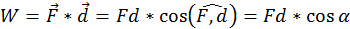In the international system of units (SI) work is measured in Joules (J). One Joule is equal to the work done by a 1 Newton force, the application point of which moves 1 meter parallel with the force and in its direction. Thus if the force that acts on the body is opposing its motion, the work is always negative.

Example 1:  Work Done by Gravity

The first instance is the work done by gravity on a falling body. Thus the work done by the gravity force is independent of the path taken by the body when falling and on how the body is moving in its fall, but is equal to the product between the weight of the body and difference of level (H) between the initial and final points: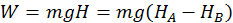Example 2:  Work Done by a Spring

The second instance is the work done by elastic forces when a spring is stretched or compressed. The elastic force always depends on the displacement from the equilibrium position, so that it is not constant (You may recall the formula for this: F(x) = -kx).  Because the two quantities are directly related, calculating the total work requires an integral.  You can do so with the following formula: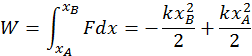Both the elastic force and the gravitational force are capable of doing work, the amount of which depends only on the initial and final points of the body motion.  The energy that is responsible for these types of work is called potential energy and the source of the work done by a body is the energy that it has.

1. Transformations & Conservation of Energy

When work is done on a body, the body state changes between points A and B (that the body is moved). For this reason, the energy a body has is a physical quantity that depends on the state of the body and each of the points A and B correspond to a certain different energy. Mechanical energy has two parts: kinetic energy and potential energy.

Kinetic Energy

The energy that a body has that is due to its motion is called kinetic energy.  We define the kinetic energy of a body that is in translational motion with speed as being equal to half of the product between the mass of the body and its speed squared  (K.E. = 1/2 mv^2).  We can derive this by starting from the equation of the uniform accelerated motion using the following methods:

Start with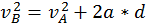and multiply everything by the mass.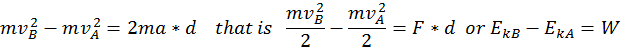Thus, when a body is in motion, it can act on a second body doing work. This work is equal to the change in Kinetic Energy, as shown in the formula above where the variation of the kinetic energy of a body is equal to the work done by the force that acts on that body.

Gravitational Potential Energy

The potential energy describing the position of the body with respect to the earth is called gravitational potential energy. The gravitational potential energy is equal the mechanical work done by gravity (which we call weight) but has the opposite sign.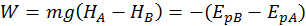A body found at a greater height has a bigger potential gravitational energy.  So, when we bring a body to a greater height, we do work on it.  Gravity is also doing work on it, but this work is negative, since it is opposing the body motion.

Elastic Potential Energy

The potential energy describing the deformation of a spring with respect to its rest position is called elastic potential energy. Again, by convention, the potential elastic energy variation is equal and contrary to the work done by the elastic force: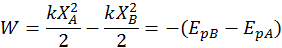Note:  a spring either compressed or stretched has positive potential energy (because elastic forces always oppose the displacement X from the equilibrium position and thus do negative work) since P.E. = 1/2kx^2.

Conservation of Total Energy

Building off the idea that the change in kinetic energy is equal to the work done, we can write the following equation: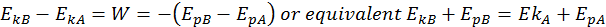This is the Law of Conservation of Total Energy.  The total energy is the sum between its potential and kinetic energies, and it always remains constant if the body moves into a conservative field of forces.

If you are interested in experimenting with conservation of total energy, there are two very nice applets on the web that let you do this. To see work and gravitational potential energy in action go to Energy State Park, to experiment with springs and potential energy go to Masses and Springs. A good physics book for college where you can learn further about work and energy can be found on Amazon here.

## College Thermodynamics Homework Help

Since we have tutors in all Thermodynamics related topics, we can provide a range of different services. Our online Thermodynamics tutors will:

• Provide specific insight for homework assignments.
• Review broad conceptual ideas and chapters.
• Simplify complex topics into digestible pieces of information.
• Answer any Thermodynamics related questions.
• Tailor instruction to fit your style of learning.

With these capabilities, our college Thermodynamics tutors will give you the tools you need to gain a comprehensive knowledge of Thermodynamics you can use in future courses.